﻿ 无人机群多目标协同主动感知的自组织映射方法
«上一篇文章快速检索 高级检索

 智能系统学报2020, Vol. 15Issue (3): 609-614  DOI: 10.11992/tis.2019080220

### 引用本文WANG Huaxian, HUA Rong, LIU Huaping, et al. Self-organizing feature map method for multi-target active perception of unmanned aerial vehicle systems[J]. CAAI Transactions on Intelligent Systems, 2020, 15(3): 609-614. DOI: 10.11992/tis.201908022.### 文章历史

1. 上海应用技术大学 电气与电子工程学院，上海 201499;
2. 清华大学 智能技术与系统国家重点实验室，北京 100084

Self-organizing feature map method for multi-target active perception of unmanned aerial vehicle systems
WANG Huaxian 1, HUA Rong 1, LIU Huaping 2, ZHAO Huailin 1, SUN Fuchun 2
1. School of Electrical and Electronics Engineering, Shanghai Institute of Technology, Shanghai 201499, China;
2. State Key Lab of Intelligent Technology and Systems, Tsinghua University, Beijing 100084, China
Abstract: It is known that the robots fitted with cameras, also sometimes called as unmanned aerial vehicles, are used to monitor inaccessible areas. The data sent by robots are analyzed through various artificial neural networks. Active vision is particularly important to cope with problems like occlusions, limited field of view, and limited resolution of the camera. In view of the active vision difficulty faced in active perception system, this paper proposes an active perception framework of heterogeneous robots based on the self-organizing feature map, a type of multi-objective active learning algorithm, and plans the shortest smooth path for the drone team to traverse all the targets. First, the multi-target active perception scene is modeled as multiple traveling salesmen problem with neighborhood. After that, the self-organizing mapping network is used to find the shortest closed-loop track for travel time, and then the track is smoothed with the third-order Bézier curve, a parametric curve. The simulation results and comparative experiments show that proposed method has better effects in the application of multi-objective active perception.
Key words: multi-robot system    active perception    traveling salesman problem    self-organizing mapping network    path planning    heterogeneous robot    Bézier curve    path smoothing

1 相关工作 1.1 主动感知

1.2 带邻域的多旅行商问题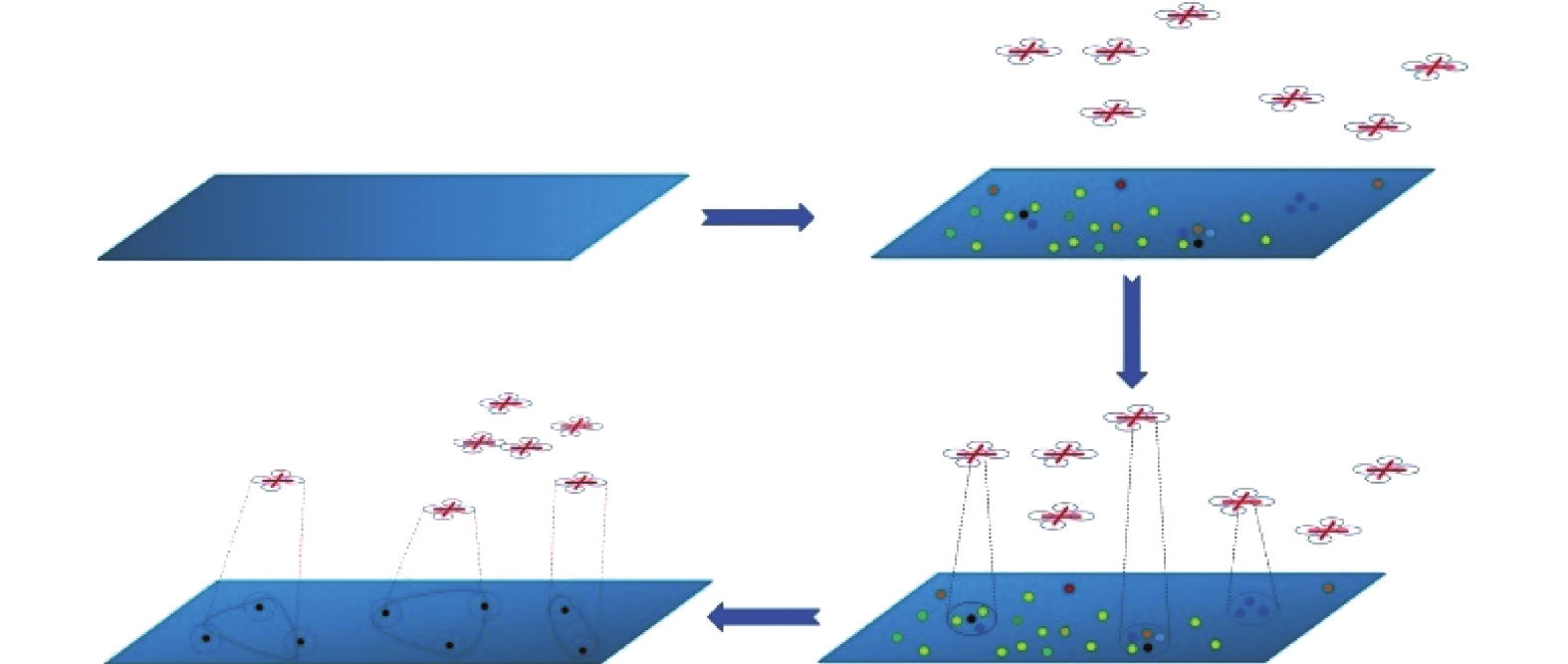Download: 图 1 多目标主动感知场景 Fig. 1 Multi-target active perception scene
1.3 自组织映射特征网络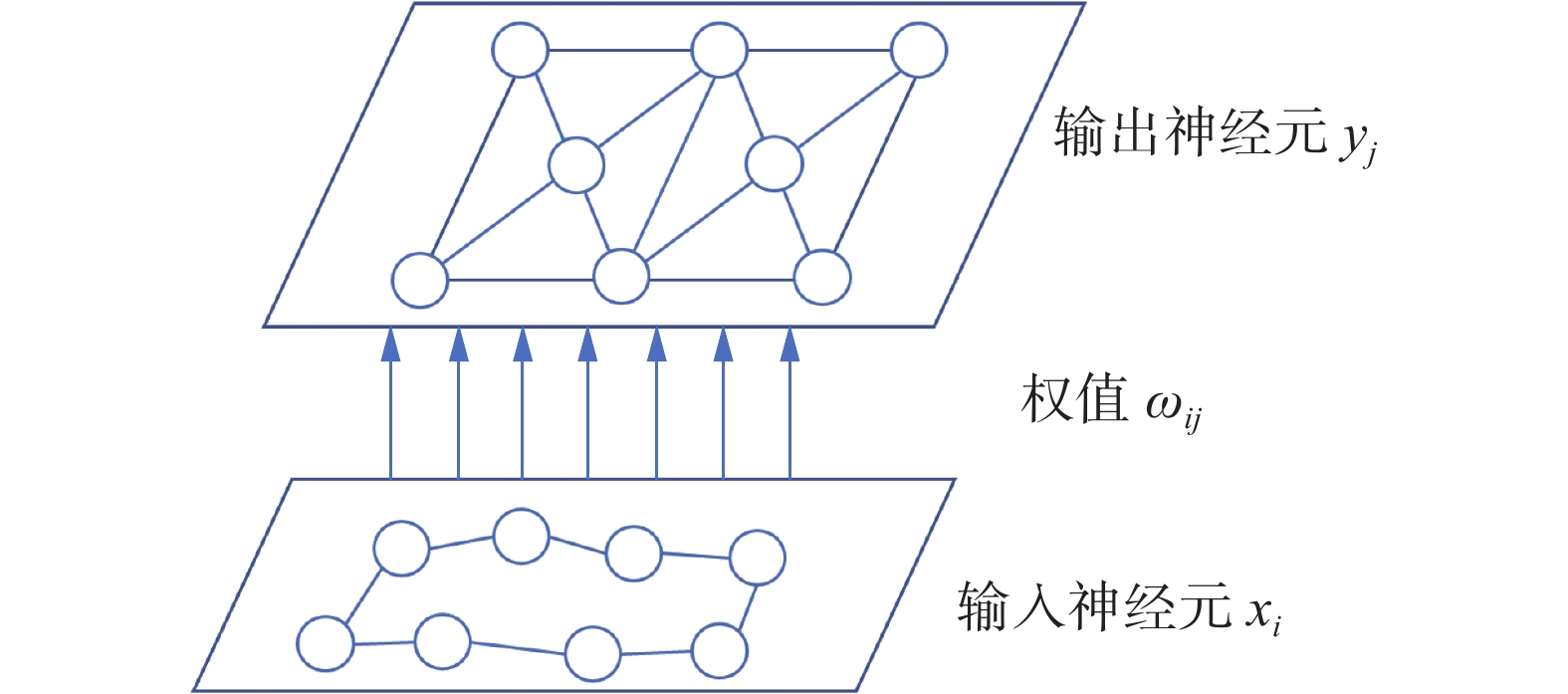Download: 图 2 典型的SOM结构 Fig. 2 Typical SOM structure diagram
2 问题建模

 $\min {\rm{mize}} T(X)=\displaystyle \sum\limits_{i=1}^{n} T\left(X_{i}\right)$

SOM规划出的平滑路径都有旅行消耗 ${c_i} \geqslant 0$ ，定义为无人机在路径 ${X_i}$ 上的时间。机器人团队有总的旅行预算时间 ${b_i} \geqslant 0$ 。当SOM为机器人团队规划出一次路径时，会计算出当前轮次的旅行消耗，选择获胜机器人 ${R}_{r}$ ，即

 $R_{r} \leftarrow \arg \min \left(\dfrac{c_{q}}{b_{q}}\right), \quad c_{i} \leqslant b_{i}。$
3 感知路径生成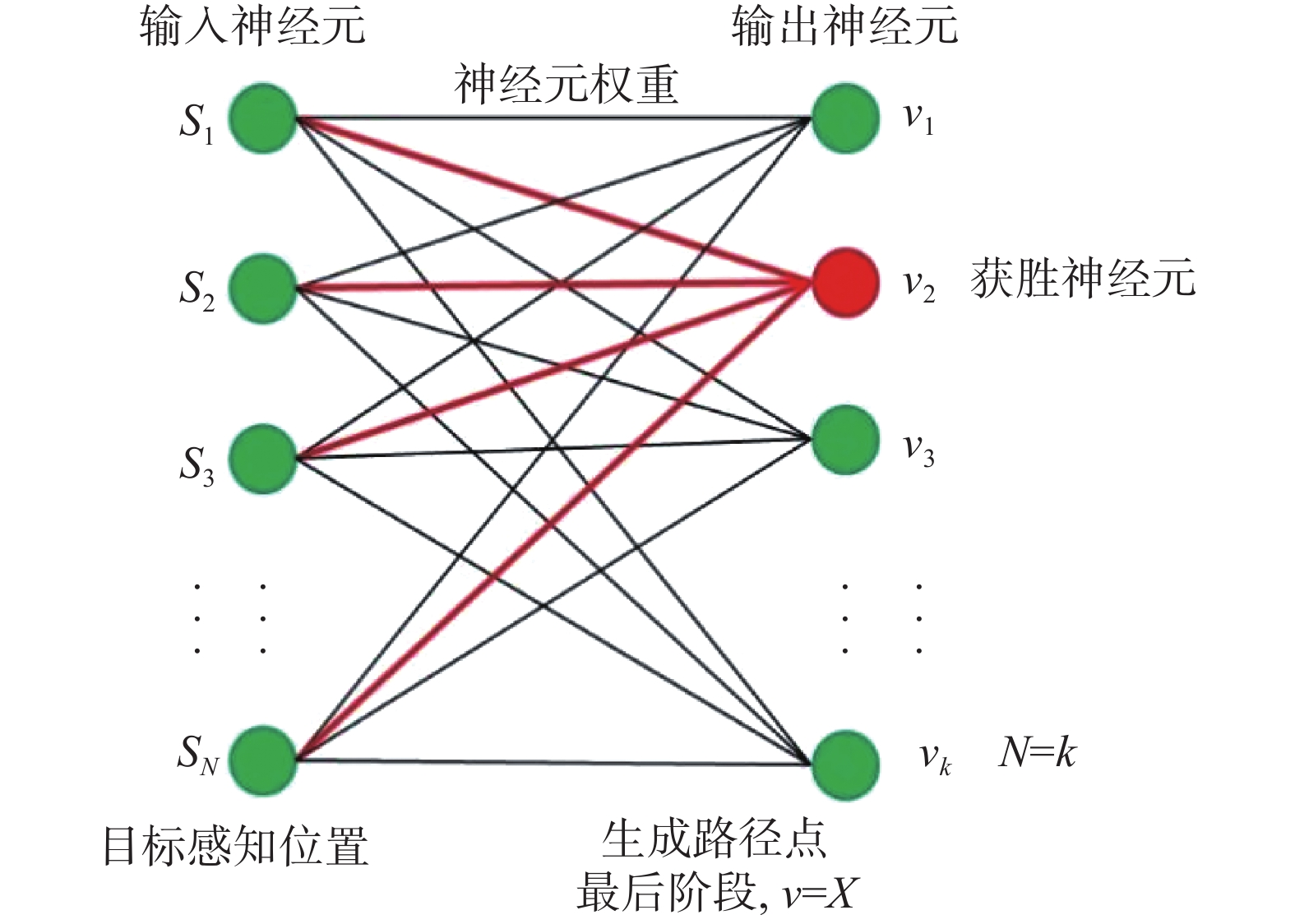Download: 图 3 SOM训练结构 Fig. 3 SOM training structure

 ${T_{j,I(x)}} = \exp \left( - \dfrac{{S_{j,I(x)}^2}}{{2{\sigma ^2}}}\right)$ (1)

 $\sigma(t)=\sigma_{0} \exp \left(-t / \tau_{\sigma}\right)$ (2)

 $\Delta \omega_{j i}=\eta(t) \cdot T_{j, I(x)}(t) \cdot\left(x_{i}-\omega_{j i}\right)$ (3)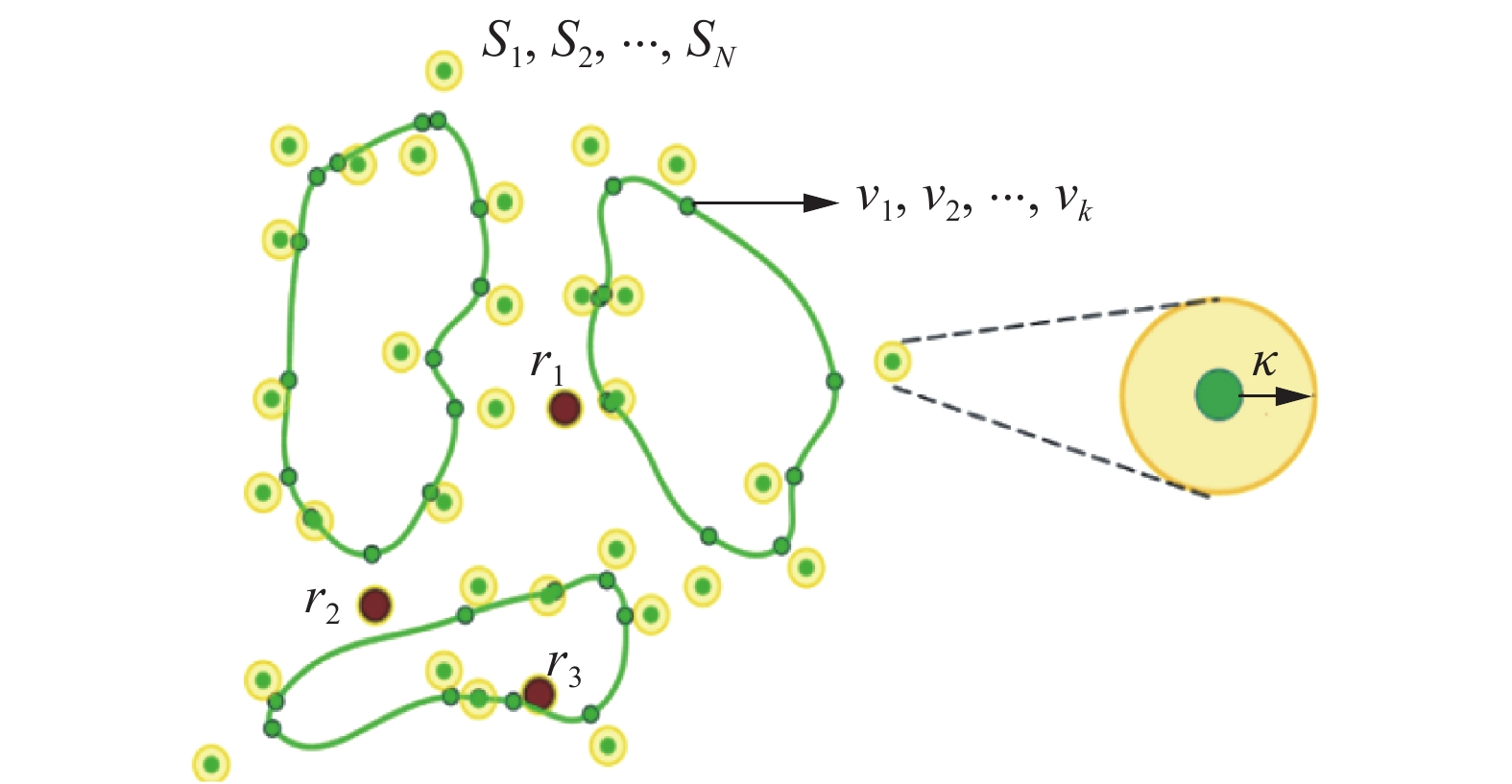Download: 图 4 SOM训练过程 Fig. 4 SOM training process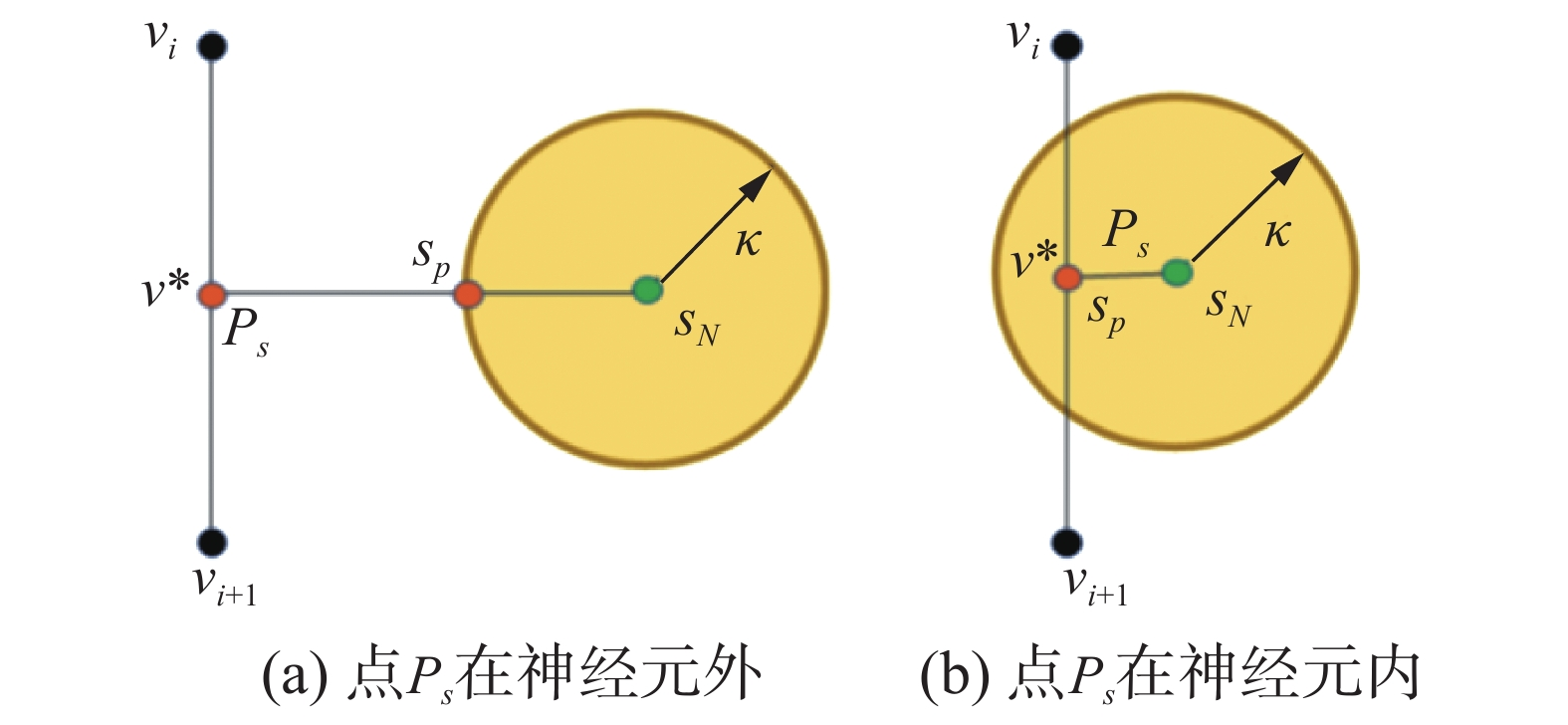Download: 图 5 获胜神经元的几何关系 Fig. 5 Geometry of the winner neuron

 $v^{\prime}=v+\mu f(\sigma, d)\left(v^{*} s_{p}-v\right)$ (4)

 $f(\sigma ,d) = \left\{ \begin{array}{l} \operatorname{e} ^\frac{{ - {d^2}}}{{{\sigma ^2}}},\;\quad d < 0.2M \\ 0,\;\quad {\text{其他}} \\ \end{array} \right.$ (5)

 $\sigma {\rm{ = }}\left( {{\rm{1 - }}\alpha } \right)\sigma$ (6)

 ${X\left(\tau \right)=B}_{0}{(1-\tau )}^{3}+3{B}_{1}\tau {(1-\tau )}^{2}+\\ 3{B}_{2}{\tau }^{2}\left(1-\tau \right)+{B}_{3}{\tau }^{3}$ (7)

 $t_a^{i - 1} = B_1^{i - 1} - B_0^{i - 1},\quad t_b^i = B_3^i - B_2^i\\$ (8)
 $l_a^{i - 1} = \left\| {t_a^{i - 1}} \right\|,\quad l_b^i = \left\| {t_b^i} \right\|$ (9)

 $l_a^{i - 1}t_b^i = l_b^it_a^{i - 1}$ (10)
4 实验结果及分析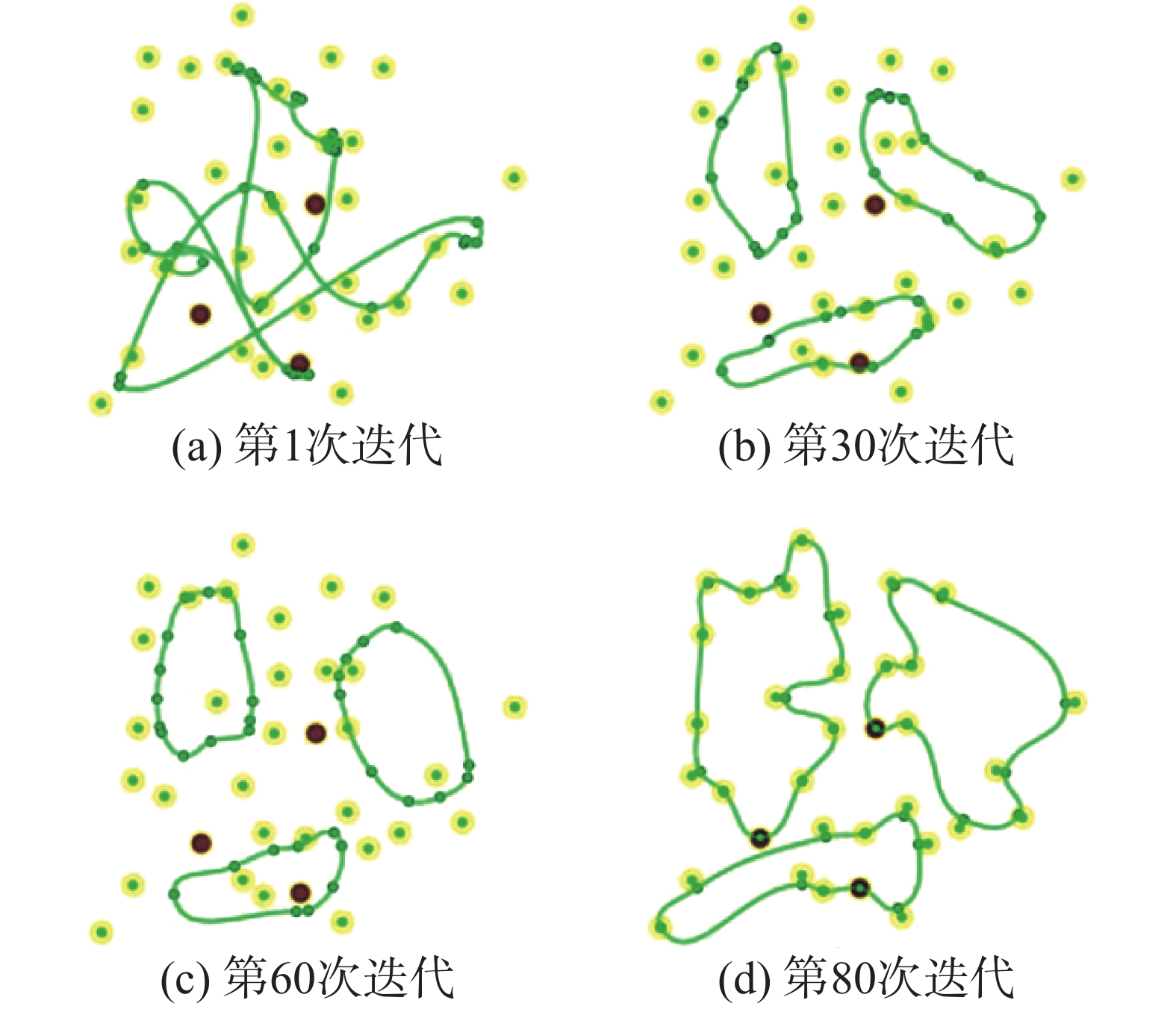Download: 图 6 SOM迭代过程 Fig. 6 SOM iterative process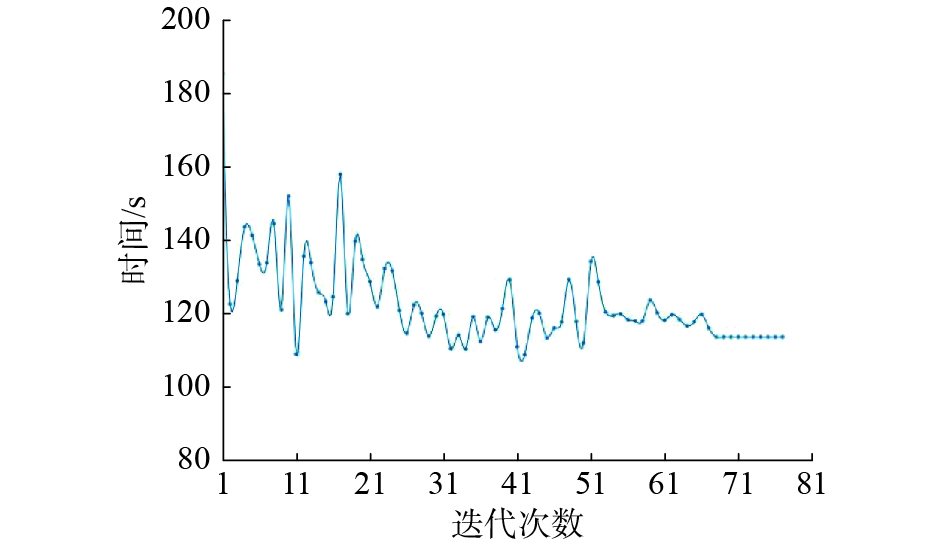Download: 图 7 单次实验的旅行时间 Fig. 7 Traveling time of a single experiment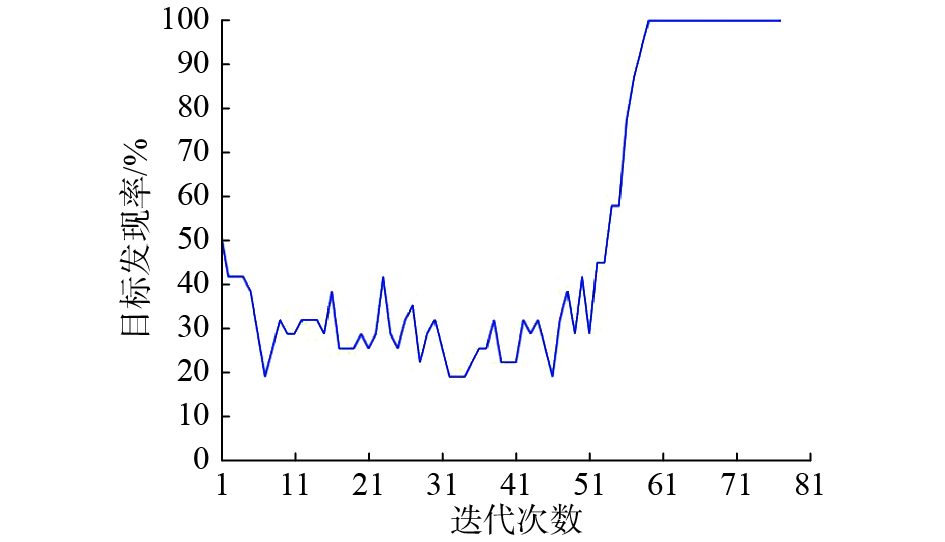Download: 图 8 单次实验的目标发现率 Fig. 8 Target discovery rate of a single experiment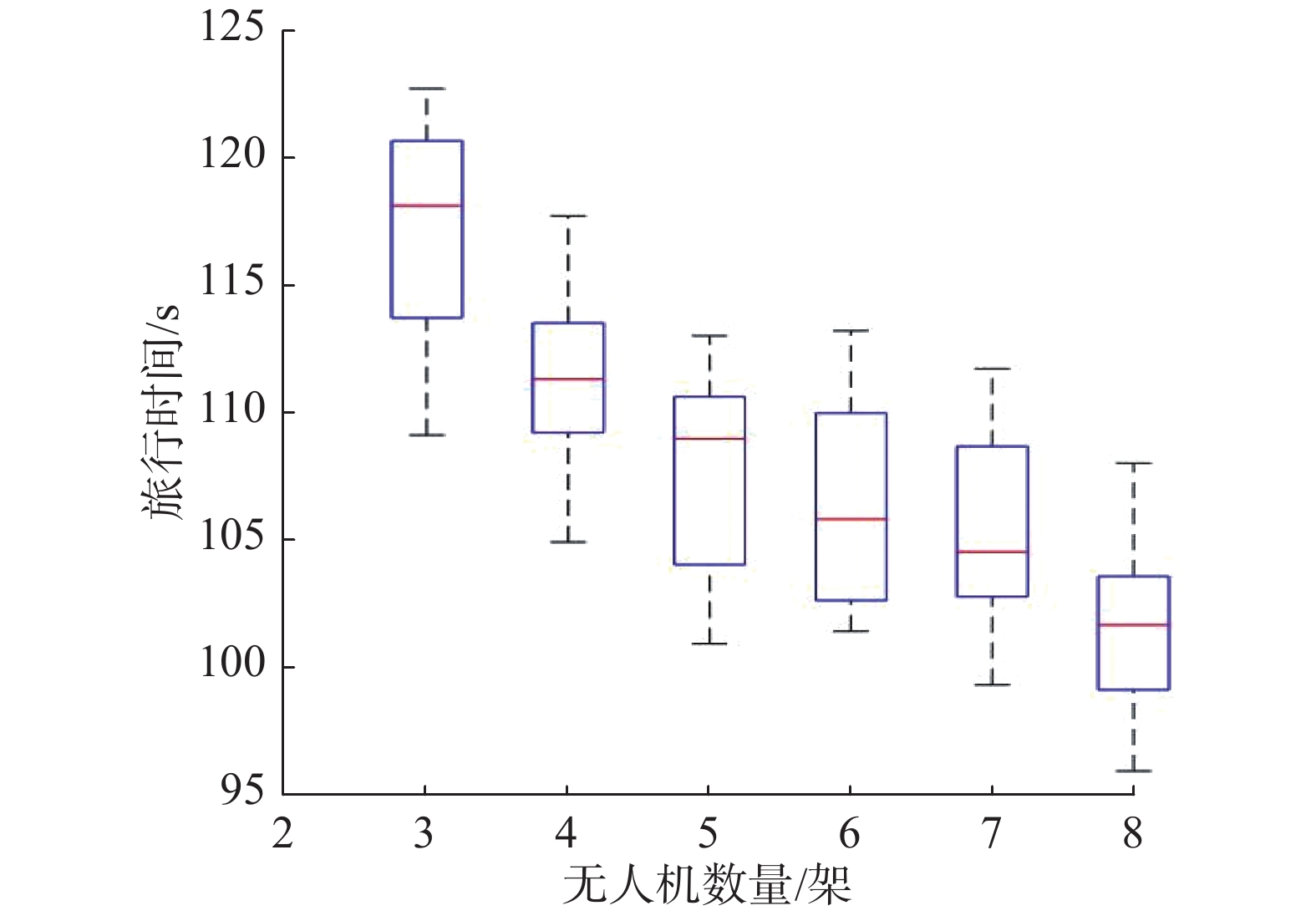Download: 图 9 无人机数量与总旅行时间的关系 Fig. 9 Relationship between the number of UAVs and the total travel time表 1 不同算法的旅行时间 Tab.1 Traveling time for different algorithms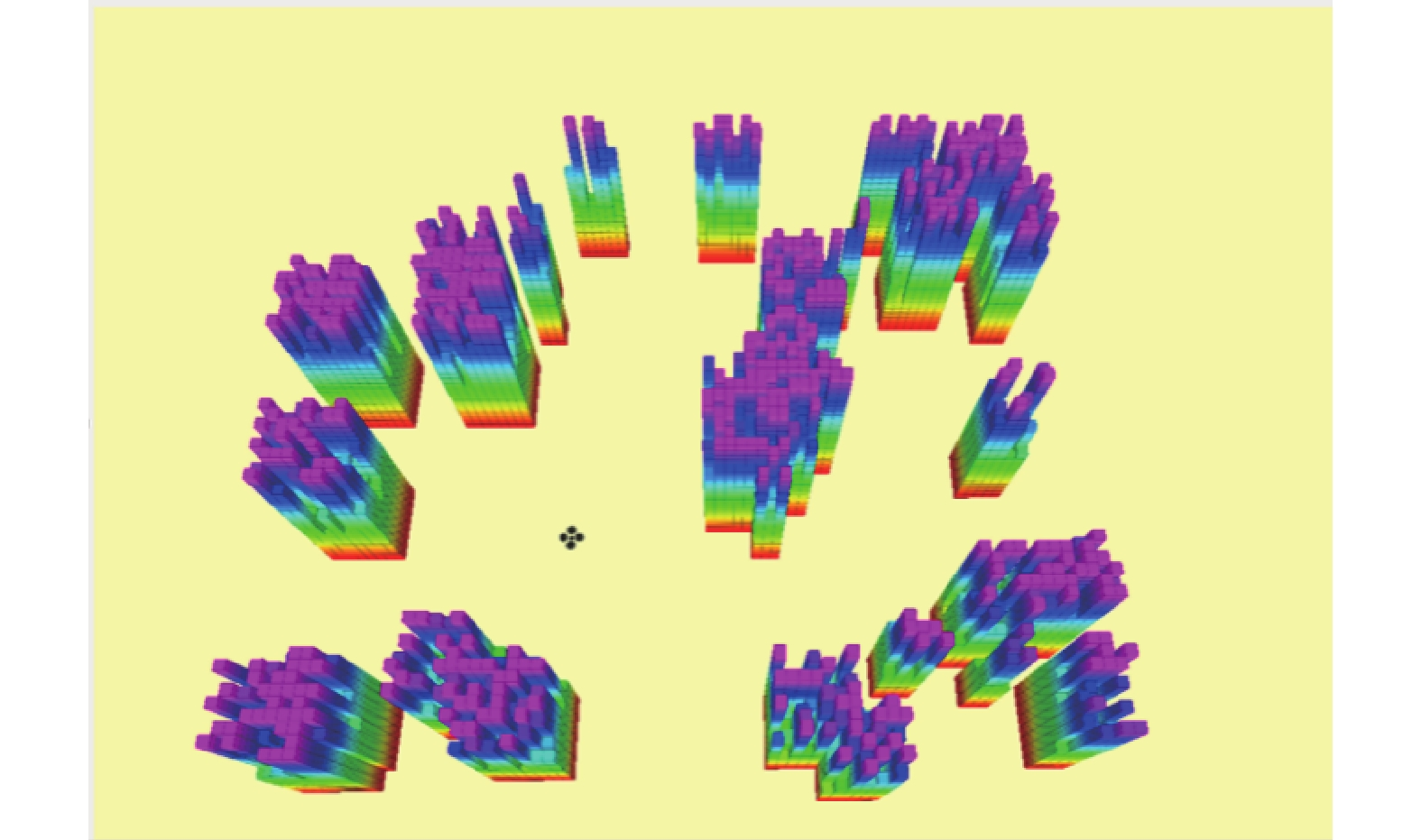Download: 图 10 搭建gazebo仿真环境 Fig. 10 Building a simulation environment in gazebo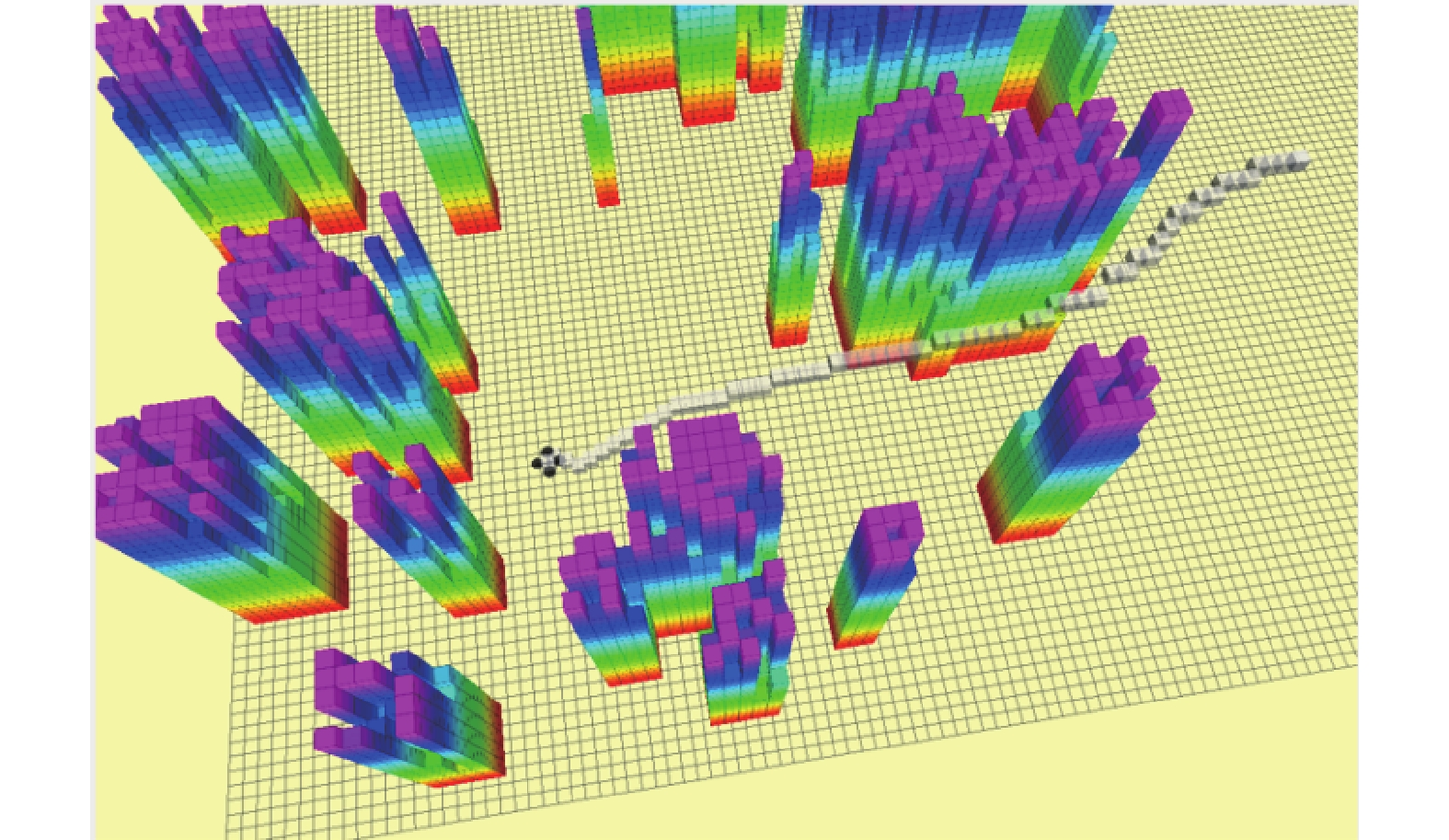Download: 图 11 gazebo中的算法测试结果 Fig. 11 Algorithm test results in gazebo
5 结束语

  ATANASOV N, LE NY J, DANIILIDIS K, et al. Decentralized active information acquisition: Theory and application to multi-robot SLAM[C]//2015 IEEE International Conference on Robotics and Automation (ICRA). Seattle, USA: 2015: 4775−4782. (0)  GIFFORD C M, WEBB R, BLEY J, et al. A novel low-cost, limited-resource approach to autonomous multi-robot exploration and mapping[J]. Robotics and autonomous systems, 2010, 58(2): 186-202. DOI:10.1016/j.robot.2009.09.014 (0)  BEST G, FAIGL J, FITCH R. Online planning for multi-robot active perception with self-organising maps[J]. Autonomous robots, 2018, 42(4): 715-738. DOI:10.1007/s10514-017-9691-4 (0)  BEST G, CLIFF O M, PATTEN T, et al. Dec-MCTS: Decentralized planning for multi-robot active perception[J]. The international journal of robotics research, 2019, 38(2/3): 316-337. (0)  FITCH R, ISLER V, TOKEKAR P, et al. Guest editorial: special issue on active perception[J]. Autonomous robots, 2018, 42(2): 175-176. DOI:10.1007/s10514-018-9695-8 (0)  CHEN Shengyong, LI Youfu, KWOK N M. Active vision in robotic systems: A survey of recent developments[J]. The international journal of robotics research, 2011, 30(11): 1343-1377. DOI:10.1177/0278364911410755 (0)  BAJCSY R. Active perception[J]. Proceedings of the IEEE, 1988, 76(8): 966-1005. DOI:10.1109/5.5968 (0)  BAJCSY R, ALOIMONOS Y, TSOTSOS J K. Revisiting active perception[J]. Autonomous robots, 2018, 42(2): 177-196. DOI:10.1007/s10514-017-9615-3 (0)  CHARROW B. Information-theoretic active perception for multi-robot teams[D]. Philadelphia, USA: University of Pennsylvania, 2015. (0)  GIBSON J J. The ecological approach to visual perception[M]. New York, USA: Psychology Press, 2013. (0)  PATTEN T, ZILLICH M, FITCH R, et al. Viewpoint evaluation for online 3-D active object classification[J]. IEEE robotics and automation letters, 2016, 1(1): 73-81. DOI:10.1109/LRA.2015.2506901 (0)  YAN Zhi, JOUANDEAU N, CHERIF A A. A survey and analysis of multi-robot coordination[J]. International journal of advanced robotic systems, 2013, 10(12): 399. DOI:10.5772/57313 (0)  SCHLOTFELDT B, THAKUR D, ATANASOV N, et al. Anytime planning for decentralized multirobot active information gathering[J]. IEEE robotics and automation letters, 2018, 3(2): 1025-1032. DOI:10.1109/LRA.2018.2794608 (0)  FAIGL J, HOLLINGER G A. Unifying multi-goal path planning for autonomous data collection[C]//IEEE/RSJ International Conference on Intelligent Robots and Systems. Chicago, USA: 2014. (0)  BEKTAS T. The multiple traveling salesman problem: an overview of formulations and solution procedures[J]. Omega, 2006, 34(3): 209-219. DOI:10.1016/j.omega.2004.10.004 (0)  MOHAJER A, BAVAGHAR M, FARROKHI H. Mobility-aware load balancing for reliable self-organization networks: multi-agent deep reinforcement learning[J]. Reliability engineering and system safety, 2020, 202: 107056. DOI:10.1016/j.ress.2020.107056 (0)  欧伟奇, 尹辉, 许宏丽, 等. 一种基于Multi-Egocentric视频运动轨迹重建的多目标跟踪算法[J]. 智能系统学报, 2019, 14(2): 246-253. OU Weiqi, YIN Hui, XU Hongli, et al. A multi-object tracking algorithm based on trajectory reconstruction on Multi-Egocentric video[J]. CAAI transactions on intelligent systems, 2019, 14(2): 246-253. DOI:10.11992/tis.201709003 (0)  李景灿, 丁世飞. 基于人工鱼群算法的孪生支持向量机[J]. 智能系统学报, 2019, 14(6): 1121-1126. LI Jingcan, DING Shifei. Twin support vector machine based on artificial fish swarm algorithm[J]. CAAI transactions on intelligent systems, 2019, 14(6): 1121-1126. DOI:10.11992/tis.201905025 (0)  张飞, 白伟, 乔耀华, 等. 基于改进D*算法的无人机室内路径规划[J]. 智能系统学报, 2019, 14(4): 662-669. ZHANG Fei, BAI Wei, QIAO Yaohua, et al. UAV indoor path planning based on improved D* algorithm[J]. CAAI transactions on intelligent systems, 2019, 14(4): 662-669. DOI:10.11992/tis.201803031 (0)  刘建华, 刘华平, 杨建国, 等. 测距式传感器同时定位与地图创建综述[J]. 智能系统学报, 2015, 10(5): 655-662. LIU Jianhua, LIU Huaping, YANG Jianguo, et al. A survey of range-only SLAM for mobile robots[J]. CAAI transactions on intelligent systems, 2015, 10(5): 655-662. DOI:10.11992/tis.201403017 (0)